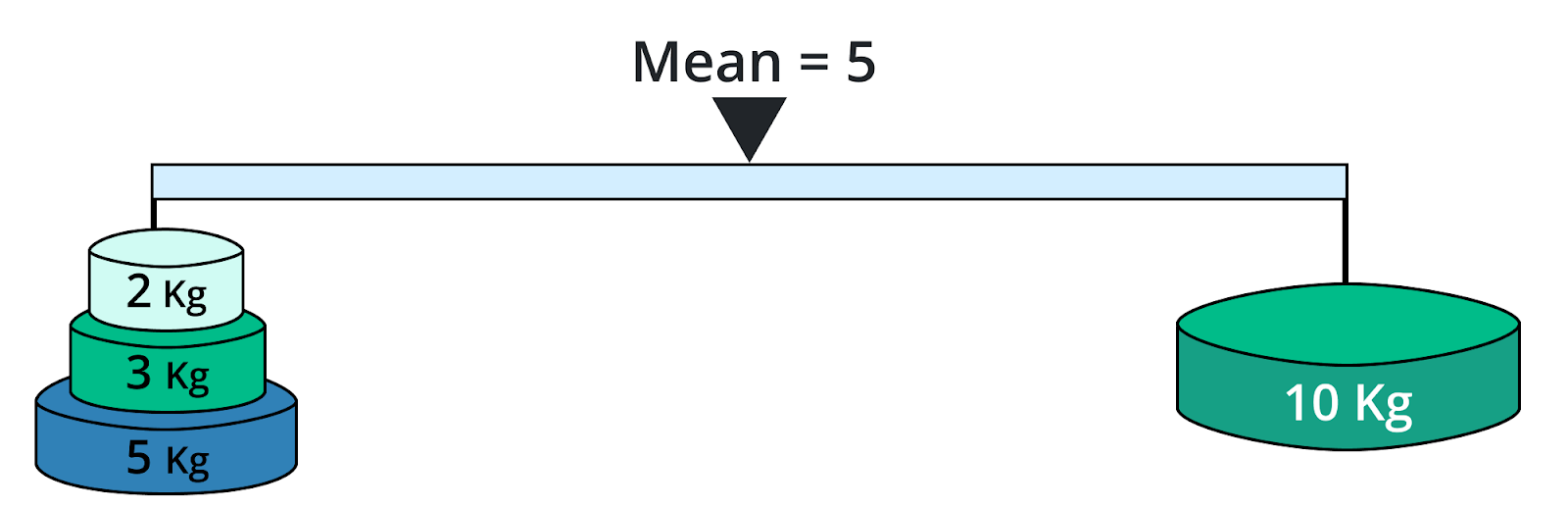# Average Calculator

To find the average, input comma-separated values and click the calculate button using average calculator

Formula:
Average = Sum of all valuesNo. of Values

Give Us Feedback

## Average calculator

Average calculator is used to find the average value (mean value) of the given data set. This calculator finds the value of the arithmetic mean with steps.

## What is average?

In mathematics, the average is the sum of numbers in the data set divided by the total numbers in the data set. Average (A.K.A mean) is a measurement of essential propensity. It tells us which number is the most common in data collection and which number best reflects all of the numbers in the data set.### Formula of Average

The formula of average to find the arithmetic mean is:

Average = Sum of values/Total number of values

Such as:

Average = (a1 +a2 +a3 +….an)/n

## How to find the average?

It is very simple to find the average of the numbers, just follow the below steps

• Add up all the numbers in the given set
• Divided by the total numbers in the given set

## How to calculate the average?

Try the average calculator above to calculate the average value of the given data set in no time. Follow the below examples to evaluate the average of the given data set manually.

Example 1

Find the average of 4,8,12,14.

Solution

Step 1: Add all the values of the given data set.

4 + 8 + 12 + 14 = 38

Step 2: Count total numbers "n" in the given data set.

n = 4

Step 3: Now take the formula of average and put the value of sum and total numbers to evaluate the average value.

Average = Sum of values/Total number of values

Average = 38/4

Average = 9.5

Example 2

Find the average of 5, -9, 8, 14, -4.

Solution

Step 1: Add all the values of the given data set.

5 + (-9) + 8 + 14 + (-4) = 14

Step 2: Count total numbers "n" in the given data set.

n = 5

Step 3: Now take the formula of average and put the value of sum and total numbers to evaluate the average value.

Average = Sum of values/Total number of values

Average = 14/5

Average = 2.8

## Some other examples of average

 Average of 15, 30, 45, 60 30 Average of 17, 29, 39, 49, 59 38.6 Average of 9, 13, 17, 27, 33, 41 23.33 Average of 35, 65, 78, 89, 107, 208, 101 97.5

### Math Tools